# Trigonometry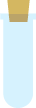Trigonometry helps you understand any topic that involves distances, angles, or waves. The trig functions (sin, cos, and tan) show up all over science and engineering.

« Back to dashboard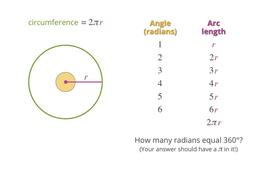What's a "radian"?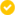Discover another way to measure angles.Radians and degreesHow to switch back and forth between units for anglesLengths of circular arcsA formula for arc length in terms of radius and angle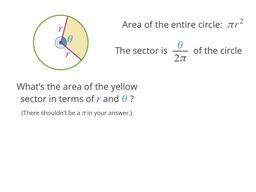SectorsLearn what a sector is, and a formula for finding its area
2. Trig functions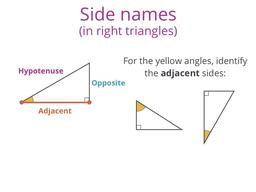Sides of right triangles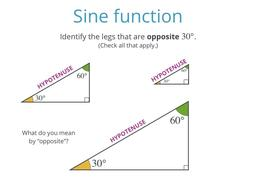Sine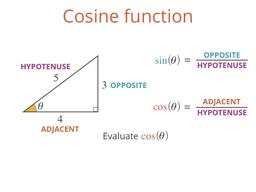CosineAlong with sine, cosine is a fundamental trig function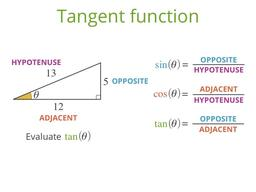TangentAnother important trig function (like sine and cosine)Components (and cops)Find legs of right triangles, and don't get pulled over!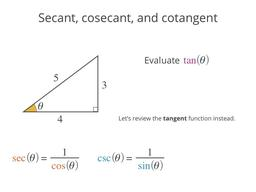Sec, Csc, and CotSecant and friends (three more trig functions)
3. Special angles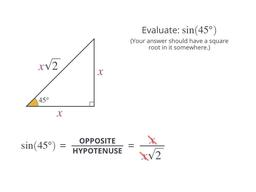45 degreesCalculate the sine, cosine, and tangent of 45 degrees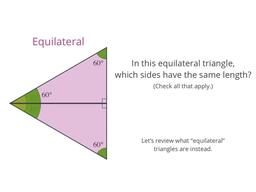30/60 degreesCalculate the sine, cosine, and tangent of 30 and 60 degrees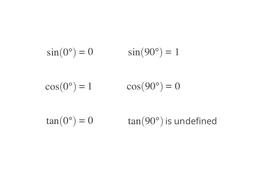0/90 degreesCalculate the sine, cosine, and tangent of 0 and 90 degrees
4. The unit circle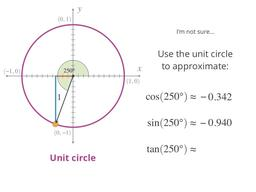Trig for larger anglesWhat's sin(250)? Use the unit circle to tackle bigger angles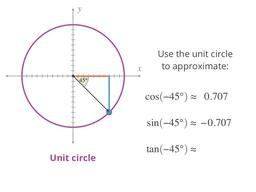Trig for negative anglesUsing the unit circle for trig with negative angles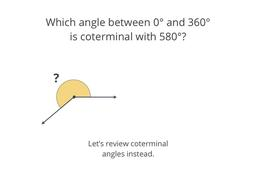Trig for coterminalsHow trig functions are related for coterminal angles
5. Inverse trig functionsInverse sineA function that takes you from side ratios to angles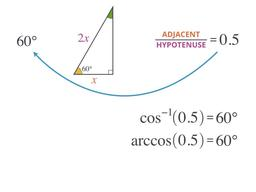Inverse cosineAnother inverse trig functionInverse tangentAnd one last inverse trig function!
6. Trig identities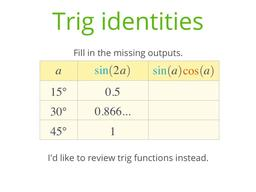Trig identitiesThe tools for simplifying trig expressions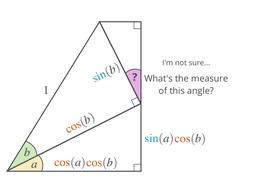Addition identitiesSimplify trig functions of sums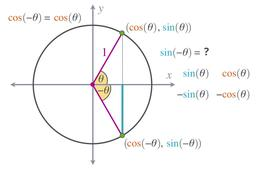Negative angle identitiesSimplify trig functions of negative anglesSubtraction identitiesSimplify trig functions of differences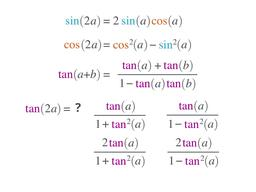Double angle identitiesTrig functions of twice an angle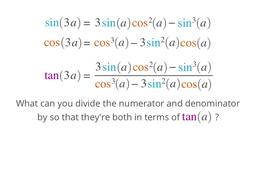Triple angle identitiesTrig functions of three times an anglePythagorean identitiesRelating squares of trig functionsHalf angle identitiesTrig functions of half an angle
7. Triangle formulasLaw of sinesRelating angles and opposite sides for any triangle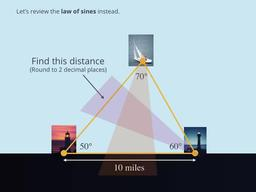TriangulationReal-world application of the law of sines to find distances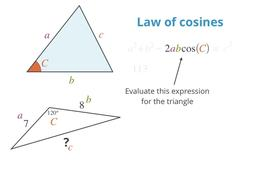Law of cosinesRelating three sides and an angle for any triangle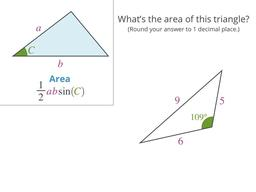Triangle area: SASFind a triangle's area using 2 sides and the angle between